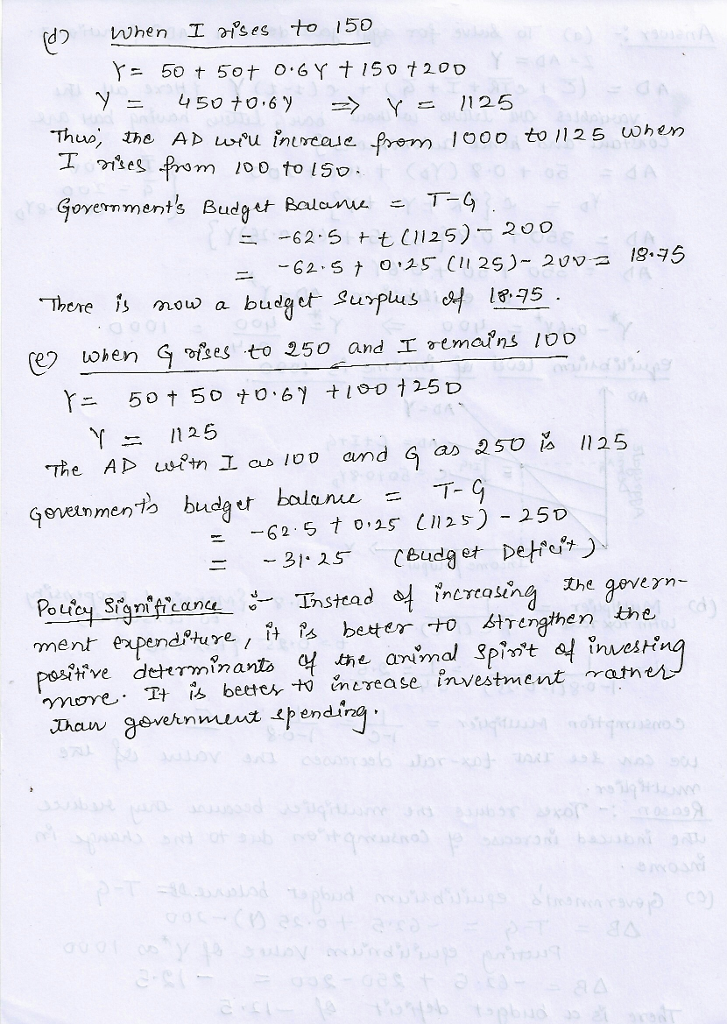# Question & Answer: Consider a closed economy (NX = 0) with a government that spends, taxes, and makes transfer payments to households. Government spending is…..

Consider a closed economy (NX = 0) with a government that spends, taxes, and makes transfer payments to households. Government spending is G = 200 and the tax function is T = −62.5 + 0.25Y (note the − sign is not a mistake; the −62.5 term includes transfer payments which are like negative taxes—they flow to households and add to disposable income—so think of T as “taxes net of transfers”). The consumption function is C = 50 + 0.8YD and investment is fixed at I = 100.
a. Solve for the aggregate demand function Z and the equilibrium level of income Y. Illustrate your answer on a diagram.
b. Calculate the value of the multiplier. Discuss how the multiplier is affected by the tax rate, t1 = 0.25. Would a higher tax rate increase or decrease the multiplier? Why?
c. What is the government’s equilibrium budget balance (surplus) (T − G)? (Hint: Use the equilibrium value of Y*).
d. If the “animal spirits” of business firms improve (confidence increases), so that I rises to 150, what happens to Y ? What happens to the budget balance? Calculate and explain.
e. What happens if the government increases its spending G to 250 (with investment back at I = 100)? Compare the effects with part d. of this question and discuss how they are similar or different. (What is the policy significance of the difference?)